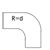Calculation of pressure drop in pipework

Select unit
 SI units US units

Please use a decimal point as the separator!

Calculation of pressure drop

 Flow rate V m³/min Nominal length L m Pipework inner diameter Id mm Absolute working pressure p bar Pressure drop Δ p bar

 The nominal length of the pipeline is calculated by adding together the length of the straight pipeline and the equivalent lengths of the fittings installed. An estimate of the equivalent lengths of the fittings can be provided using the adjacent program or accepted on an approximate basis by adding 60% onto the length of the straight pipeline, e.g. nominal length = length of straight pipeline x 1.6. The calculation is valid for smooth pipes.

Calculate the equivalent pipe length

 Piping diameter DN 25 40 50 80 100 125 150 200 250 300 400 Equivalent length [m] Number Knee bend1.5 Bend R = 2d0.3 Bend R = d0.4 Outlet direction of T-piece0.5 Branching direction of T-piece1.5 Seat valve5 Butterfly valve/ball valve0.3 Check valve2 Reduction to DN:   200.5 Equivalent pipe length of all fittings installed m